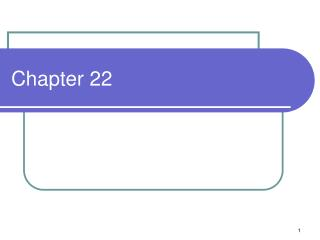DownloadDownload PresentationChapter 22

# Chapter 22

Download Presentation## Chapter 22

- - - - - - - - - - - - - - - - - - - - - - - - - - - E N D - - - - - - - - - - - - - - - - - - - - - - - - - - -
##### Presentation Transcript

1. Chapter 22

2. Flux • Number of objects passing through a surface

3. Electric Flux, F • is proportional to the number of electric field lines passing through a surface • Assumes that the surface is perpendicular to the lines • If not, then we use a cosine of the angle between them to get the components that are parallel • Mathematically:

4. Simple Cases A A A q F=EAcosq F=EA E cosq F=0 E E E

5. From S to • A S represents a sum over a large a collection of objects • Integration is also a sum over a collection of infinitesimally small objects, in our case, small areas, dA • So

6. Gauss’s Law • The field lines emitted by a charge are proportional to the size of the charge. • Therefore, the electric field must be proportional to the size of the charge • In order to count the field lines, we must enclose the charges in some geometrical surface (one that we choose)

7. Mathematically Charge enclosed within bounding limits of this closed surface integral

8. Fluxes, Fluxes, Fluxes

9. 3 Shapes • Sphere • Cylinder • Pillbox

10. Sphere • When to use: around spherical objects (duh!) and point charges • Hey! What if an object is not one of these objects? • Closed surface integral yields: • r is the radius of the geometrical object that you are creating

11. Sphere Example What if you had a sphere of radius, b, which contained a material whose charge density depend on the radius, for example, r=Ar2where A is a constant with appropriate units? At r=b, both of these expressions should be equal

12. Cylinder • When to use: around cylindrical objects and line charges • Closed surface integral yields: • r is the radius of the geometrical object that you are creating and L is the length of the cylinder L

13. Cylinder Example What if you had an infinitely long line of charge with a linear charge density, l?

14. Pillbox • When to use: around flat surfaces and sheets of charge • Closed surface integral yields: • A is the area of the pillbox

15. Charge Isolated Conductor in Electrostatic Equilibrium • If excess charge is placed on an isolated conductor, the charge resides on the surface. Why? • If there is an E-field inside the conductor then it would exert forces on the free electrons which would then be in motion. This is NOT electrostatic. • Therefore, if there is no E-field inside, then, by Gauss’s Law, the charge enclosed inside must be zero • If the charges are not on the outside, you are only left with the surface • A caveat to this is that E-field lines must be perpendicular to the surface else free charges would move.

16. A E +++++++++++++++++++++++++++++++++++++++++++++ E Electric field on an infinitely large sheet of charge

17. Electric field on a conducting sheet A E +++++++++++++++++++++++++++++++++++++++++++++ So a conductor has 2x the electric field strength as the infinite sheet of charge

18. A differential view of Gauss’s Law • Recall the Divergence of a field of vectors How much the vector diverges around a given point Div=+large Div=0

19. Divergence Theorem (aka Gauss’s Thm or Green’s Thm) Suspiciously like LHS of Gauss’s Law A place of high divergence is like a faucet Bounded surface of some region Sum of the faucets in a volume = Sum of the water going thru the surface

20. Div(E) So how the E-field spreads out from a point depends on the amount of charge density at that point